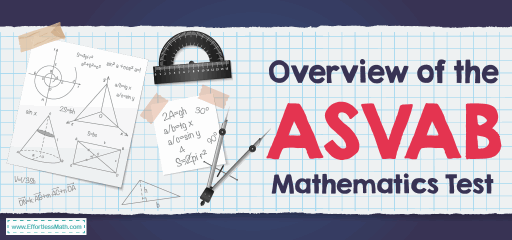# Overview of the ASVAB Mathematics TestThe Armed Services Vocational Aptitude Battery or ASVAB test is a multiple-choice format qualification test used by the military to assess your membership eligibility. However, this is not just used to determine if you qualify for registration.

The ASVAB consists of a series of subtests. Each subtest will contain a certain number of multiple-choice questions, and you will have limits time for each section are from 10 to 36 minutes. The whole exam takes three hours. Categories for subtests are:

• Arithmetic Reasoning (AR)
• Word Knowledge (WK)
• Paragraph Comprehension (PC)
• Mathematics Knowledge (MK)
• Electronics Information (EI)
• Assembling Objects (AO)
• Auto and Shop Information (AS)
• Mechanical Comprehension (MC)
• General Science (GS)

Your mathematics skills will be tested through ASVAB Mathematics Knowledge and Arithmetic Reasoning subtests, but strong mathematics skills can also be useful in some other subtests like Electronics Information.

## What does the ASVAB Math test cover?

The math section of the ASVAB test across two different elements of math knowledge includes:

### 1- Mathematics Knowledge (MK)

The Mathematics Knowledge (MK)section of the test covers basic high school-level mathematics rules, terms, and concepts. The P & P-ASVAB has 25 questions with a 24-minute time limit while CAT-ASVAB has 16 questions with a 20-minute limit for the finish of the section.

The questions in this section of the test are in the form of math problems. The key to studying this section is to understand the basic math concepts and how they work! You should have a good understanding of topics common in high school algebra courses, such as:

• Order of operations
• Simple geometry (circles, triangles, rectangles)
• Exponents
• Factorials
• Fractions, percentages, and interest
• Different types of numbers (rational numbers, real numbers, integers, prime numbers)

### 2- Arithmetic Reasoning (AR)

The Arithmetic Reasoning (AR) section tests your ability to put your math skills to work by solving some basic word problems. The P & P-ASVAB has 30 questions that give you 36 minutes while CAT-ASVAB has 16 questions that you have 39 minutes to complete the section.

Just answering each question for a minute or two can make this part of the test a little scary. But if you know how to approach the questions in this section, you will not have any problems. To solve these word problems, you must:

• Identify the question you are being asked.
• Rewrite that question as a mathematical equation.
• List the information you need to know to solve the problem.
• List each step you need to take to solve the problem.

## Is the ASVAB math hard?

The ASVAB mathematics is a difficult area for many, but with patience and logic, it can be easy and even enjoyable!

## Do you get a formula sheet on the ASVAB?

If you want to improve your score in the ASVAB math section, you will need essential formulas.

## Can you use the calculator on the ASVAB test?

No, you do not get to bring a calculator to use while taking the ASVAB test.

## How is the ASVAB Test Scored?

Test candidates are given a standard score for each of the sub-tests. Standard scores are derived from your raw scores. A standard score is a score that is statistically derived and typically varies from 30 to 70 for each subtest.

## More from Effortless Math for ASVAB Test …

### Looking for a FREE online ASVAB Math course to help you learn all ASVAB Mathematics topics?

Check out The Ultimate ASVAB Math Course.

### How to ace the math subtests of the ASVAB test?

Read the following post to get the answer: Ace the Math Subtests of the ASVAB Test

## Have any questions about the ASVAB Test?

### What people say about "Overview of the ASVAB Mathematics Test - Effortless Math: We Help Students Learn to LOVE Mathematics"?

No one replied yet.

X
52% OFF

Limited time only!

Save Over 52%

SAVE \$40

It was \$76.99 now it is \$36.99